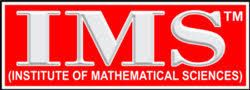# Exam Pattern: Graduate Aptitude Test in Engineering (GATE) – Mathematics

## Graduate Aptitude Test in Engineering (GATE) – Mathematics

### Pattern of Question Papers

The examination for the papers with codes AE, AG, AR, GG, MN and TF will be carried out ONLINE using computers where the candidates will be required to enter the answer for each question using mouse. For all other papers, the candidates will have to mark the correct choice on an Optical Response Sheet (ORS) by darkening the appropriate bubble against each question.

In the ONLINE papers, the question paper will consist of questions of multiple choice type and numerical answer type. For multiple choice type questions, each question will have four choices for the answer. For numerical answer type questions, each question will have a number as the answer and choices will not be given.

##### GATE contain questions of four different types in various papers:
• Multiple choice questions carrying 1 or 2 marks each.
• Common data questions, where two successive questions use the same set of input data.
• Numerical answer questions, where the answer is a number, to be entered by the candidate.

In all the papers, there will be a total of 65 questions carrying 100 marks, out of which 10 questions carrying 15 marks in General Aptitude (GA) are compulsory.

In the papers bearing the codes AE, AG, BT, CE, CH, CS, EC, EE, GG, IN, ME, MN, MT, PI, TF and XE, the Engineering Mathematics will carry 15 % of the total marks, the General Aptitude section will carry 15 % of the total marks and the remaining 70 % of the total marks is devoted to the subject of the paper.

In the papers bearing the codes AR, CY, GG, MA, PH and XL, the General Aptitude section will carry 15 % of the total marks and the remaining 85 % of the total marks is devoted to the subject of the paper.

### Pattern of Question Papers

General Aptitude (GA) Section:  In all papers, GA questions are of multiple choice type, and carry a total of 15 marks. The GA section includes 5 questions carrying 1 mark each (sub-total 5 marks) and 5 questions carrying 2-marks each (sub-total 10 marks)

Question papers other than GG, XE and XL:  These papers would contain 25 multiple choice questions carrying one mark each (sub-total 25 marks) and 30 multiple choice questions carrying two marks each (sub-total 60 marks). Out of these, two pairs of questions would be common data questions, and two pairs of questions would be linked-answer questions. In the ONLINE papers, the question paper will consist of questions of multiple choice type and numerical answer type. For multiple choice type questions, each question will have four choices for the answer. For numerical answer type questions, each question will have a number as the answer and choices will not be given.

GG (Geology and Geophysics) Paper:  Apart from the General Aptitude (GA) section, the question paper consists of two parts: Part A and Part B. Part A is common for all candidates. Part B contains two sections: Section 1 (Geology) and Section 2 (Geo-physics). Candidates will have to attempt questions in Part A and either Section 1 or Section 2 in Part B.

Part A consists of 25 multiple choice questions carrying 1-mark each (sub-total 25 marks). Each section in Part B (Section 1 and Section 2) consists of 30 multiple choice questions carrying 2-marks each (sub-total 60 marks). Out of these, two pairs of questions would be common data questions, and two pairs of questions would be linked-answer questions.

XE Paper (Engineering Sciences):  In XE paper, Engineering Mathematics section (Section A) is compulsory. This section contains 11 multiple choice questions carrying a total of 15 marks: 7 questions carrying 1-mark each (sub-total 7 marks), and 4 questions carrying 2-marks each (sub-total 8 marks).

Each of the other sections of the XE paper (Sections B through G) contains 22 questions carrying a total of 35 marks: 9 questions carrying 1-mark each (sub-total 9 marks) and 13 questions carrying 2-marks each (sub-total 26 marks). Out of the 2-mark questions, 2 pairs are common data questions and 1 pair is linked answer questions.

XL Paper (Life Sciences):  In XL paper, Chemistry section (Section H) is compulsory. This section contains 15 multiple choice questions carrying a total of 25 marks: 5 questions carrying 1 mark each (sub-total 5 marks) and 10 questions carrying 2-marks each (sub-total 20 marks). Out of the 2-mark questions, 1 pair is common data questions, and 1 pair is linked answer questions.

Each of the other sections of the XL paper (Sections I through M) contains 20 multiple choice questions carrying a total of 30 marks: 10 questions carrying 1-mark each (sub-total 10 marks) and 10 questions carrying 2-marks each (sub-total 20 marks).